Next: The p' function Up: Auxiliary operations Previous: Multiplication of a stream

## Addition or subtraction of one from stream

It is possible to define two simple functions to add or subtract the number one from the number represented by a from a stream of signed binary digits (provided the stream represents a number in a suitable interval). Let us define these functions as follows:

These functions may be implemented as follows:

 = f(0::x') = f(1::x') = g(0::x') = g(1::x') =

The proof of correctness for such definitions is as follows. Let us consider the function f applied to a numeral x. The proof for the function g is similar. We use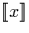to denote the number represented by the numeral x. We examine the three cases in turn:

Input x starts with the digit 1:
If the input x starts with a one we know that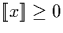. Hence we can output an infinite stream of ones (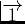) by the function definition.
Input x starts with the digit :
If the input is x is of the form (0::x'), we know that x represents the value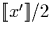. Now, using the properties described in section 3.6.2:

Input x starts with the digit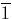:
If the input x is of the form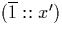, we can simply add the number one at the first digit and return (1::x'):Next: The p' function Up: Auxiliary operations Previous: Multiplication of a stream
Martin Escardo
5/11/2000